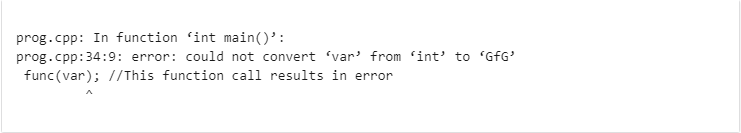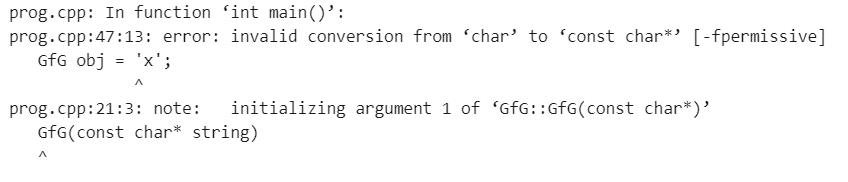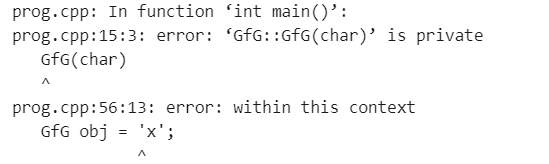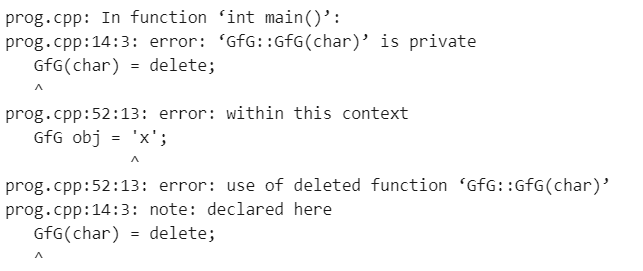Open in App
Not now

# Problem with Single Argument Constructor in C++ and How to solve it

• Last Updated : 25 Feb, 2022

In C++, if a class has a constructor which can be called with a single argument, then this constructor becomes a conversion constructor because such a constructor allows automatic conversion to the class being constructed.

Problem:
Whenever there is a constructor with a single argument and there is a function that takes an argument of the same class type but when this function is called using an argument type same as that of the constructor, in that case, the function gets called successfully. This is because the argument is implicitly converted to the class type by the constructor. The argument gets passed to the constructor and then the function gets executed. This is something we would not expect.

Below is the C++ program that demonstrates the above problem:

## C++

 `// C++ program to implement ` `// the above approach ` `#include ` `using` `namespace` `std; ` ` `  `// Defining the class ` `class` `GfG { ` `    ``int` `data; ` ` `  `public``: ` `    ``// Constructor with single parameter ` `    ``GfG(``int` `a) ` `        ``: data(a) ` `    ``{ ` `    ``} ` ` `  `    ``// Default constructor ` `    ``GfG() {} ` ` `  `    ``// Defining function to print ` `    ``// the value of data member ` `    ``void` `display() ` `    ``{ ` `        ``cout << ``"Value of data is: "` `<< data; ` `    ``} ` `}; ` ` `  `// User-defined function that takes ` `// object of class GfG as argument ` `void` `func(GfG o) ` `{ ` `    ``o.display(); ` `} ` ` `  `// Driver code ` `int` `main() ` `{ ` `    ``int` `var = 10; ` ` `  `    ``// Function gets called even if ` `    ``// int type argument is passed ` `    ``func(var); ` `}`

Output

`Value of data is: 10`

Explanation:
In the above code, there is a user-defined constructor that takes an argument of type GFG (class type) and there is a function that also takes an argument of class type. When there is an attempt to invoke the function by passing int type parameter, in this case, the function is called successfully. This happens because of the user-defined constructor. The int value passed to the function is implicitly converted to class type and the data member gets initialized with the value of the passed parameter (var).

Solution:
In order to avoid this problem of implicit conversion is to make the constructor explicit. Below is the C++ program to demonstrate the solution to the implicit conversion problem-

## C++

 `// C++ program to implement ` `// the above approach ` `#include ` `using` `namespace` `std; ` ` `  `// Defining the class ` `class` `GfG { ` `    ``int` `data; ` ` `  `public``: ` `    ``// Constructor with single parameter ` `    ``// declared explicit ` `    ``explicit` `GfG(``int` `a) ` `        ``: data(a) ` `    ``{ ` `    ``} ` ` `  `    ``// Default constructor ` `    ``GfG() {} ` ` `  `    ``// Function to print value ` `    ``// of data member ` `    ``void` `display() ` `    ``{ ` `        ``cout << ``"Value of data is: "` `<< data; ` `    ``} ` `}; ` ` `  `// User-defined function that takes ` `// object of class GfG as argument ` `void` `func(GfG o) ` `{ ` `    ``o.display(); ` `} ` ` `  `// Driver code ` `int` `main() ` `{ ` `    ``int` `var = 10; ` ` `  `    ``// This function call results ` `    ``// in error ` `    ``func(var); ` `}`

Output:Drawback:
This approach, however, has some drawbacks. What if in the same program the user really wants to convert int data type to class data type and assign an int value to an object of the class. The following assignment will result in an error-

```int var = 10;
GfG obj = var;  // This will result in error```

To solve this problem you can explicitly convert var to GfG and then assign it to obj.

`GfG obj = (GfG) var; // This works fine`

Below is the C++ program to implement the above approach-

## C++

 `// C++ program to implement ` `// the above approach ` `#include ` `using` `namespace` `std; ` ` `  `// Defining the class ` `class` `GfG { ` `    ``int` `data; ` ` `  `public``: ` `    ``// Constructor with single parameter ` `    ``// declared explicit ` `    ``explicit` `GfG(``int` `a) ` `        ``: data(a) ` `    ``{ ` `    ``} ` ` `  `    ``// Default constructor ` `    ``GfG() {} ` ` `  `    ``// Function to print value ` `    ``// of data member ` `    ``void` `display() ` `    ``{ ` `        ``cout << ``"Value of data is: "` `<< data; ` `    ``} ` `}; ` ` `  `// User-defined function that takes ` `// object of class GfG as argument ` `void` `func(GfG o) ` `{ ` `    ``o.display(); ` `} ` ` `  `// Driver code ` `int` `main() ` `{ ` `    ``int` `var = 10; ` ` `  `    ``// Explicitly converting var to ` `    ``// class (GfG) type ` `    ``GfG obj = (GfG)var; ` ` `  `    ``// Calling function with the ` `    ``// converted variable obj ` `    ``func(obj); ` `}`

Output

`Value of data is: 10`

Let’s consider one more example and discuss what happens when explicit will also not work.

## C++

 `// C++ program to implement ` `// the above approach ` `#include ` `using` `namespace` `std; ` ` `  `// Defining the class ` `class` `GfG { ` `private``: ` `    ``string str; ` ` `  `public``: ` `    ``// Constructor with single parameter ` `    ``// declared explicit ` `    ``GfG(``int` `a) ` `    ``{ ` `        ``str.resize(a); ` `    ``} ` ` `  `    ``// Default constructor ` `    ``GfG(``const` `char``* string) ` `    ``{ ` `        ``str = string; ` `    ``} ` ` `  `    ``// Function to print value ` `    ``// of data member ` `    ``void` `display() ` `    ``{ ` `        ``cout << ``"String is: "` `<< str << ``"\n"``; ` `    ``} ` `}; ` ` `  `// User-defined function that takes ` `// object of class GfG as argument ` `void` `func(GfG o) ` `{ ` `    ``o.display(); ` `} ` ` `  `// Driver code ` `int` `main() ` `{ ` `    ``// This line will compile and ` `    ``// will use GFG(int) ` `    ``GfG obj = ``'x'``; ` ` `  `    ``// Calling function with the ` `    ``// converted variable obj ` `    ``func(obj); ` `    ``return` `0; ` `}`

Output

`String is:`

Explanation:
In the above example, the user is trying to initialize a string with a char value but char is a part of the integer family, so the compile will use the constructor GfG(int) to implicitly convert char to GfG. This will produce unexpected results.
As discussed above one of the solutions to this problem is to use the keyword explicit.

## C++

 `// C++ program to implement ` `// the above approach ` `#include ` `using` `namespace` `std; ` ` `  `// Defining the class ` `class` `GfG { ` `private``: ` `    ``string str; ` ` `  `public``: ` `    ``// Constructor with single parameter ` `    ``// declared explicit ` `    ``explicit` `GfG(``int` `a) ` `    ``{ ` `        ``str.resize(a); ` `    ``} ` ` `  `    ``// Default constructor ` `    ``GfG(``const` `char``* string) ` `    ``{ ` `        ``str = string; ` `    ``} ` ` `  `    ``// Function to print value ` `    ``// of data member ` `    ``void` `display() ` `    ``{ ` `        ``cout << ``"String is: "` `<< str << ``"\n"``; ` `    ``} ` `}; ` ` `  `// User-defined function that takes ` `// object of class GfG as argument ` `void` `func(GfG o) ` `{ ` `    ``o.display(); ` `} ` ` `  `// Driver code ` `int` `main() ` `{ ` `    ``// Compile-time error since ` `    ``// GfG(int) is explicit, so ` `    ``// nothing will match. ` `    ``GfG obj = ``'x'``; ` ` `  `    ``// Calling function with the ` `    ``// converted variable obj ` `    ``func(obj); ` `    ``return` `0; ` `}`

Output:Explanation:
The above program will not compile, since GfG(int) was made explicit, and an appropriate converting constructor could not be found to implicitly convert ‘x’ to GfG. Please note that explicit keywords can only disallow implicit conversions, typecasting cannot be avoided using the explicit keyword as discussed above.

The delete keyword

One partial solution to the above problem is to create a private constructor GfG(char). Below is the C++ program to implement this concept:

## C++

 `// C++ program to implement ` `// the above approach ` `#include ` `using` `namespace` `std; ` ` `  `// Defining the class ` `class` `GfG { ` `private``: ` `    ``string str; ` ` `  `    ``// Objects of type char cannot ` `    ``// be constructed from outside ` `    ``// the class ` `    ``GfG(``char``) ` `    ``{ ` `    ``} ` ` `  `public``: ` `    ``// Constructor with single parameter ` `    ``// declared explicit ` `    ``explicit` `GfG(``int` `a) ` `    ``{ ` `        ``str.resize(a); ` `    ``} ` ` `  `    ``// Default constructor ` `    ``GfG(``const` `char``* string) ` `    ``{ ` `        ``str = string; ` `    ``} ` ` `  `    ``// Function to print value ` `    ``// of data member ` `    ``void` `display() ` `    ``{ ` `        ``cout << ``"String is: "` `<< str << ``"\n"``; ` `    ``} ` `}; ` ` `  `// User-defined function that takes ` `// object of class GfG as argument ` `void` `func(GfG o) ` `{ ` `    ``o.display(); ` `} ` ` `  `// Driver code ` `int` `main() ` `{ ` `    ``// Compile-time error since ` `    ``// GfG(char) is private ` `    ``GfG obj = ``'x'``; ` ` `  `    ``// Calling function with the ` `    ``// converted variable obj ` `    ``func(obj); ` `    ``return` `0; ` `}`

Output:Explanation:
In the above code, GfG(char) constructor is made private. This has prevented access to the constructor from outside the class but it can still be used inside the class. The solution to this problem is to use the delete keyword.

Delete Keyword:
Below is the C++ program to implement the concept of delete keyword:

## C++

 `// C++ program to implement ` `// the above approach ` `#include ` `using` `namespace` `std; ` ` `  `// Defining the class ` `class` `GfG { ` `private``: ` `    ``string str; ` ` `  `    ``// Any use of this constructor ` `    ``// is an error ` `    ``GfG(``char``) = ``delete``; ` ` `  `public``: ` `    ``// Constructor with single parameter ` `    ``// declared explicit ` `    ``explicit` `GfG(``int` `a) ` `    ``{ ` `        ``str.resize(a); ` `    ``} ` ` `  `    ``// Default constructor ` `    ``GfG(``const` `char``* string) ` `    ``{ ` `        ``str = string; ` `    ``} ` ` `  `    ``// Function to print value ` `    ``// of data member ` `    ``void` `display() ` `    ``{ ` `        ``cout << ``"String is: "` `<< str << ``"\n"``; ` `    ``} ` `}; ` ` `  `// User-defined function that takes ` `// object of class GfG as argument ` `void` `func(GfG o) ` `{ ` `    ``o.display(); ` `} ` ` `  `// Driver code ` `int` `main() ` `{ ` `    ``// Compile-time error since ` `    ``// GfG(char) is deleted ` `    ``GfG obj = ``'x'``; ` ` `  `    ``// Calling function with the ` `    ``// converted variable obj ` `    ``func(obj); ` `    ``return` `0; ` `}`

Output:Explanation:
When a function is deleted, any use of that function is a compile-time error.

My Personal Notes arrow_drop_up
Related Articles